Next: Conversion of the Black-Scholes Up: Solution of the Black Previous: Boundary Condition

# Derivation of the Black-Scholes equation

A neutral hedge equity is constructed by selling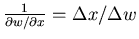call options at price w(x,t), so that the net equity invested is(1)

A change in x by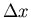accompanied by a change in w by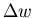then gives no change in the equity(2)

In writing the Black-Scholes equation, we will find the value of the price of the call option w(x,t) necessary to allow the hedge equity to grow at the same rate as investing the equity value in an interest account or instrument at the fixed interest rate r per day so that(3)

The Taylor expansion for the the changeis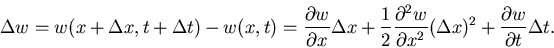(4)

It is now assumed that the variance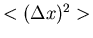comes from a random walk in the fractional price, and is therefore proportional to, giving(5)

v2 is the variance per unit time, or the variance rate.

Putting Eq. 4 and Eq. 5 into Eq. 3, cancelling the, dividing byand multiplying by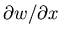gives the Black-Scholes equation(6)Next: Conversion of the Black-Scholes Up: Solution of the Black Previous: Boundary Condition
Dennis Silverman
1999-05-20